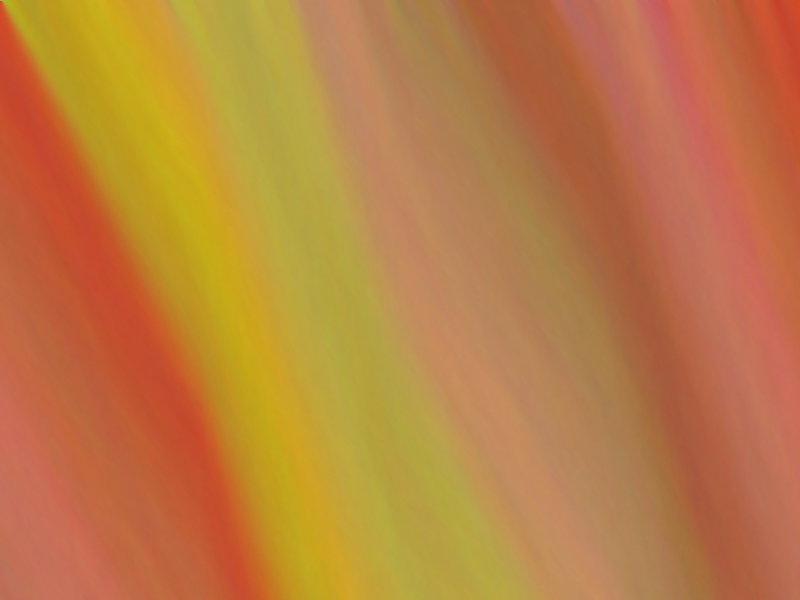A little randomness can yield remarkably organic images. The JavaScript snippet below randomly assigns colours to pixels, row-by-row starting at the top, such that the colour is similar to that of other pixels close to it.

### Try the Random Canvas generator yourself.

Remember: Each new image you generate has NEVER been created nor seen before. If you like it, then drag it to your desktop! If you make a really nice one, or if you like this, leave a comment.

Press the buttons to generate a new image. Drag images to your desktop to save.

your browser does not support the canvas tag### The JavaScript snippet

I conceived the idea and wrote the snippet in 2012 and both are released under a CC 3.0 Attribution Non-Commercial license.

function newDrawing(cwidth,cheight){
var c=document.getElementById("myCanvas");
var ctx=c.getContext("2d");
var id=ctx.createImageData(cwidth,cheight);

var i,iw4 = id.width*4, iw2 = id.width*2, iw = id.width;
id.data=Math.random()*255;
id.data=Math.random()*255;
id.data=Math.random()*255;
id.data=255;
var dir,mv;
var j;

//change this to change the amount you move in each colour
var amount = 10;

for(i = 4; i < id.height*iw4;i+=4){
//alpha
id.data[i+3]=255;
for(j=0;j<3;j++){
if(Math.random() <= 0.5){dir = -1;} else{ dir = 1;}
if(Math.random() > 0.95) mv = 1; else mv = 0;
id.data[i+j]= id.data[i+j-4] + amount*dir*mv;
//Math.max(0, Math.min( 255, id.data[i+j-4] + (0.5 + Math.random())*dir ) );
if(i>iw4+4){
if(i%iw2 > iw || Math.random() > 0.6){
id.data[i+j] = ( id.data[i+j]+ id.data[i+j-iw4+4]+ id.data[i+j-iw4-4]+ id.data[i+j-iw4])/4 + Math.random() - 0.5;
}
else
{
id.data[i+j] = ( id.data[i+j]+ id.data[i+j-iw4+4]+ id.data[i+j-iw4-4]+ id.data[i+j-iw4-4])/4 + Math.random() - 0.5;
}

} else if(i >= iw2+iw && i < iw4 && Math.random() > 0.99){ //in the first row we want to come back to the first colour
id.data[i+j] = ((iw4-i)*id.data[i+j] + (i-iw2-iw)*id.data[j])/iw + Math.random() - 0.5;

}
}
}
ctx.putImageData(id,0,0,0,0,cwidth,cheight);
var img = c.toDataURL("image/png");
// window.location = img;
var imagepng = document.getElementById("image");
imagepng.src = img;
var button = document.getElementById("save");
button.onclick = function () { window.location.href = imagepng.src.replace('image/png','image/octet-stream');};
}


### How the Random Canvas works

Think of a digital image as a grid of pixels, and each pixel has a colourIn this image, each pixel is a similar colour to the one that is left of it (or the rightmost one in the row above). How do we get similar colours?To get from to we subtracted 2 from red, added 2 to green, and subtracted 2 from blue.

To get the whole image, we started by colouring the top left pixel and then we chose a similar colour for the next pixel by adding or subtracting a small amount of each colour, and so on , , , until we coloured all the pixels, row by row, ending with the last one at the bottom right .

We randomly decide whether to subtract or add a bit of red, blue and green, to make the next pixel a random colour similar to the last one.

But that isn’t quite the end of the story, is it. After all, the pixels generated by the buttons below are similar colours to the ones above and below themselves, not just beside. If we only did what I described, the results would look like this:We fix this hideous behaviour by taking taking the average of two colours. After we have done the first row, we take our random choice of colour, and change it to the average of its own colour with that of the pixel in the row above.We are almost done! You might have noticed that there are some wavy patterns that can’t be created by merely taking the average with the pixel above. These are done by taking averages with pixels above left, or above right, depending on how far along the row we are. They are just details that don’t really change the main idea.